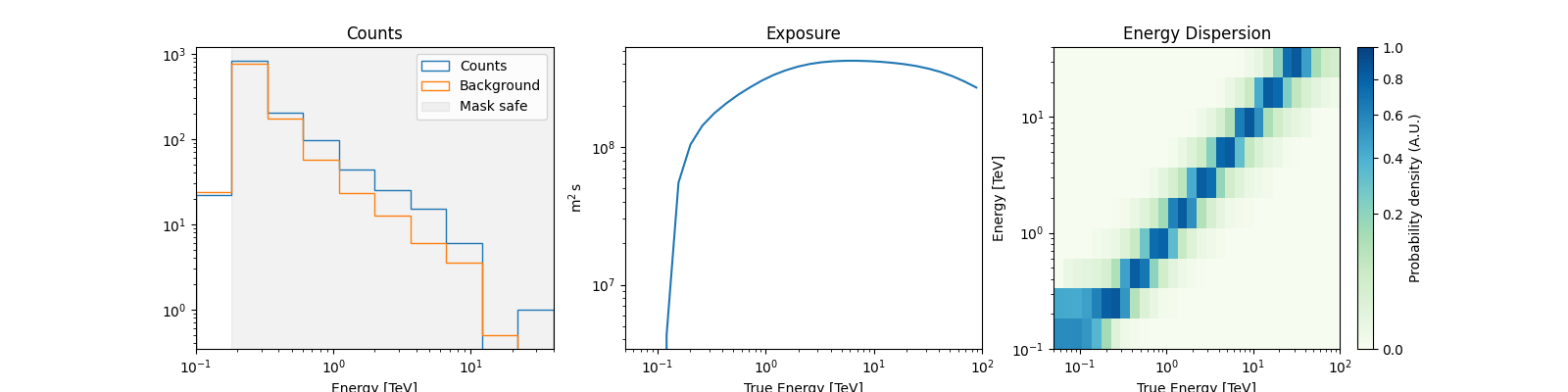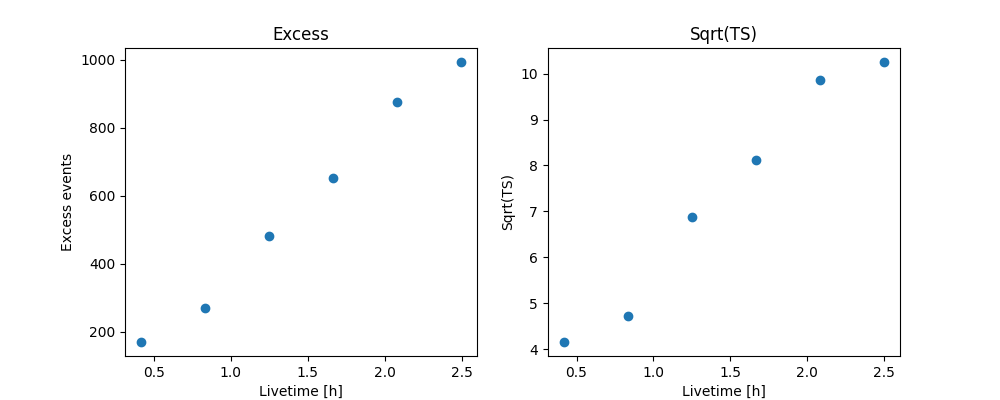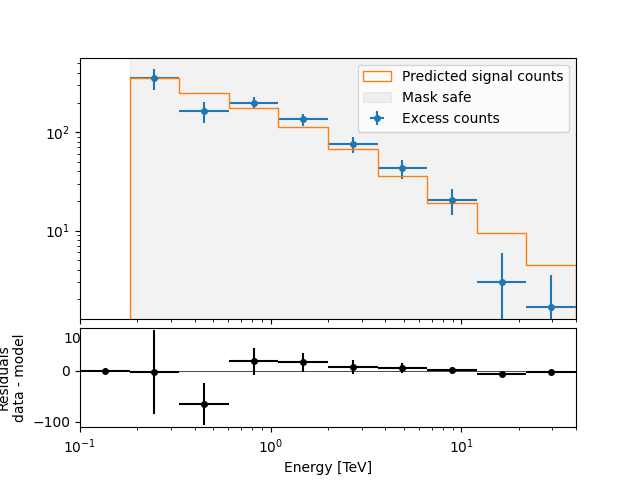# Spectral analysis of extended sources#

Perform a spectral analysis of an extended source.

## Prerequisites#

• Understanding of spectral analysis techniques in classical Cherenkov astronomy.

• Understanding the basic data reduction and modeling/fitting processes with the gammapy library API as shown in the tutorial Low level API

## Context#

Many VHE sources in the Galaxy are extended. Studying them with a 1D spectral analysis is more complex than studying point sources. One often has to use complex (i.e. non circular) regions and more importantly, one has to take into account the fact that the instrument response is non uniform over the selectred region. A typical example is given by the supernova remnant RX J1713-3935 which is nearly 1 degree in diameter. See the following article.

Objective: Measure the spectrum of RX J1713-3945 in a 1 degree region fully enclosing it.

## Proposed approach#

We have seen in the general presentation of the spectrum extraction for point sources (see Spectral analysis tutorial) that Gammapy uses specific datasets makers to first produce reduced spectral data and then to extract OFF measurements with reflected background techniques: the `SpectrumDatasetMaker` and the `ReflectedRegionsBackgroundMaker`. However if the flag `use_region_center` is not set to `False`, the former simply computes the reduced IRFs at the center of the ON region (assumed to be circular).

This is no longer valid for extended sources. To be able to compute average responses in the ON region, we can set `use_region_center=False` with the `SpectrumDatasetMaker`, in which case the values of the IRFs are averaged over the entire region.

In summary we have to:

Here, we will use the RX J1713-3945 observations from the H.E.S.S. first public test data release. The tutorial is implemented with the intermediate level API.

## Setup#

```import astropy.units as u
from astropy.coordinates import Angle, SkyCoord
from regions import CircleSkyRegion

# %matplotlib inline
import matplotlib.pyplot as plt
from IPython.display import display
from gammapy.data import DataStore
from gammapy.datasets import Datasets, SpectrumDataset
from gammapy.makers import (
ReflectedRegionsBackgroundMaker,
SpectrumDatasetMaker,
)
from gammapy.maps import MapAxis, RegionGeom
from gammapy.modeling import Fit
from gammapy.modeling.models import PowerLawSpectralModel, SkyModel
```

## Check setup#

```from gammapy.utils.check import check_tutorials_setup

check_tutorials_setup()
```
```System:

python_executable      : /home/runner/work/gammapy-docs/gammapy-docs/gammapy/.tox/build_docs/bin/python
python_version         : 3.9.16
machine                : x86_64
system                 : Linux

Gammapy package:

version                : 1.0.1
path                   : /home/runner/work/gammapy-docs/gammapy-docs/gammapy/.tox/build_docs/lib/python3.9/site-packages/gammapy

Other packages:

numpy                  : 1.24.2
scipy                  : 1.10.1
astropy                : 5.2.1
regions                : 0.7
click                  : 8.1.3
yaml                   : 6.0
IPython                : 8.11.0
jupyterlab             : not installed
matplotlib             : 3.7.1
pandas                 : not installed
healpy                 : 1.16.2
iminuit                : 2.21.0
sherpa                 : 4.15.0
naima                  : 0.10.0
emcee                  : 3.1.4
corner                 : 2.2.1

Gammapy environment variables:

GAMMAPY_DATA           : /home/runner/work/gammapy-docs/gammapy-docs/gammapy-datasets/1.0.1
```

## Select the data#

We first set the datastore and retrieve a few observations from our source.

```datastore = DataStore.from_dir("\$GAMMAPY_DATA/hess-dl3-dr1/")
obs_ids = [20326, 20327, 20349, 20350, 20396, 20397]
# In case you want to use all RX J1713 data in the HESS DR1
# other_ids=[20421, 20422, 20517, 20518, 20519, 20521, 20898, 20899, 20900]

observations = datastore.get_observations(obs_ids)
```

## Prepare the datasets creation#

### Select the ON region#

Here we take a simple 1 degree circular region because it fits well with the morphology of RX J1713-3945. More complex regions could be used e.g. `EllipseSkyRegion` or `RectangleSkyRegion`.

```target_position = SkyCoord(347.3, -0.5, unit="deg", frame="galactic")
```

### Define the geometries#

This part is especially important. - We have to define first energy axes. They define the axes of the resulting `SpectrumDatasetOnOff`. In particular, we have to be careful to the true energy axis: it has to cover a larger range than the reconstructed energy one. - Then we define the region geometry itself from the on region.

```# The binning of the final spectrum is defined here.
energy_axis = MapAxis.from_energy_bounds(0.1, 40.0, 10, unit="TeV")

# Reduced IRFs are defined in true energy (i.e. not measured energy).
energy_axis_true = MapAxis.from_energy_bounds(
0.05, 100, 30, unit="TeV", name="energy_true"
)

geom = RegionGeom(on_region, axes=[energy_axis])
```

### Create the makers#

First we instantiate the target `SpectrumDataset`.

```dataset_empty = SpectrumDataset.create(
geom=geom,
energy_axis_true=energy_axis_true,
)
```

Now we create its associated maker. Here we need to produce, counts, exposure and edisp (energy dispersion) entries. PSF and IRF background are not needed, therefore we don’t compute them.

IMPORTANT: Note that `use_region_center` is set to `False`. This is necessary so that the `SpectrumDatasetMaker` considers the whole region in the IRF computation and not only the center.

```maker = SpectrumDatasetMaker(
selection=["counts", "exposure", "edisp"], use_region_center=False
)
```

Now we create the OFF background maker for the spectra. If we have an exclusion region, we have to pass it here. We also define the safe range maker.

```bkg_maker = ReflectedRegionsBackgroundMaker()
```

## Perform the data reduction loop.#

We can now run over selected observations. For each of them, we: - create the `SpectrumDataset` - Compute the OFF via the reflected background method and create a `SpectrumDatasetOnOff` object - Run the safe mask maker on it - Add the `SpectrumDatasetOnOff` to the list.

```datasets = Datasets()

for obs in observations:
# A SpectrumDataset is filled in this geometry
dataset = maker.run(dataset_empty.copy(name=f"obs-{obs.obs_id}"), obs)

# Compute OFF
dataset = bkg_maker.run(dataset, obs)

# Append dataset to the list
datasets.append(dataset)

display(datasets.meta_table)
```
```/home/runner/work/gammapy-docs/gammapy-docs/gammapy/.tox/build_docs/lib/python3.9/site-packages/gammapy/maps/geom.py:48: RuntimeWarning: invalid value encountered in cast
p_idx = np.rint(p).astype(int)
/home/runner/work/gammapy-docs/gammapy-docs/gammapy/.tox/build_docs/lib/python3.9/site-packages/gammapy/maps/geom.py:48: RuntimeWarning: invalid value encountered in cast
p_idx = np.rint(p).astype(int)
/home/runner/work/gammapy-docs/gammapy-docs/gammapy/.tox/build_docs/lib/python3.9/site-packages/gammapy/maps/geom.py:48: RuntimeWarning: invalid value encountered in cast
p_idx = np.rint(p).astype(int)
/home/runner/work/gammapy-docs/gammapy-docs/gammapy/.tox/build_docs/lib/python3.9/site-packages/gammapy/maps/geom.py:48: RuntimeWarning: invalid value encountered in cast
p_idx = np.rint(p).astype(int)
/home/runner/work/gammapy-docs/gammapy-docs/gammapy/.tox/build_docs/lib/python3.9/site-packages/gammapy/maps/geom.py:48: RuntimeWarning: invalid value encountered in cast
p_idx = np.rint(p).astype(int)
/home/runner/work/gammapy-docs/gammapy-docs/gammapy/.tox/build_docs/lib/python3.9/site-packages/gammapy/maps/geom.py:48: RuntimeWarning: invalid value encountered in cast
p_idx = np.rint(p).astype(int)
/home/runner/work/gammapy-docs/gammapy-docs/gammapy/.tox/build_docs/lib/python3.9/site-packages/gammapy/maps/geom.py:48: RuntimeWarning: invalid value encountered in cast
p_idx = np.rint(p).astype(int)
/home/runner/work/gammapy-docs/gammapy-docs/gammapy/.tox/build_docs/lib/python3.9/site-packages/gammapy/maps/geom.py:48: RuntimeWarning: invalid value encountered in cast
p_idx = np.rint(p).astype(int)
/home/runner/work/gammapy-docs/gammapy-docs/gammapy/.tox/build_docs/lib/python3.9/site-packages/gammapy/maps/geom.py:48: RuntimeWarning: invalid value encountered in cast
p_idx = np.rint(p).astype(int)
/home/runner/work/gammapy-docs/gammapy-docs/gammapy/.tox/build_docs/lib/python3.9/site-packages/gammapy/maps/geom.py:48: RuntimeWarning: invalid value encountered in cast
p_idx = np.rint(p).astype(int)
/home/runner/work/gammapy-docs/gammapy-docs/gammapy/.tox/build_docs/lib/python3.9/site-packages/gammapy/maps/geom.py:48: RuntimeWarning: invalid value encountered in cast
p_idx = np.rint(p).astype(int)
/home/runner/work/gammapy-docs/gammapy-docs/gammapy/.tox/build_docs/lib/python3.9/site-packages/gammapy/maps/geom.py:48: RuntimeWarning: invalid value encountered in cast
p_idx = np.rint(p).astype(int)
NAME           TYPE         TELESCOP ...      RA_PNT          DEC_PNT
...       deg              deg
--------- -------------------- -------- ... --------------- -----------------
obs-20326 SpectrumDatasetOnOff     HESS ... 259.29851667325  -39.762222222222
obs-20327 SpectrumDatasetOnOff     HESS ... 257.47731666009  -39.762222222222
obs-20349 SpectrumDatasetOnOff     HESS ... 259.29851667325  -39.762222222222
obs-20350 SpectrumDatasetOnOff     HESS ... 257.47731666009  -39.762222222222
obs-20396 SpectrumDatasetOnOff     HESS ... 258.38791666667 -39.0622222341429
obs-20397 SpectrumDatasetOnOff     HESS ... 258.38791666667 -40.4622222103011
```

## Explore the results#

We can peek at the content of the spectrum datasets

```datasets.peek()
```### Cumulative excess and signficance#

Finally, we can look at cumulative significance and number of excesses. This is done with the `info_table` method of `Datasets`.

```info_table = datasets.info_table(cumulative=True)

display(info_table)
```
```  name  counts      excess     ...   acceptance_off         alpha
...
------- ------ --------------- ... ----------------- -------------------
stacked   1216           170.5 ...              18.0                 0.5
stacked   2339           270.5 ...              18.0                 0.5
stacked   3521           480.5 ...              18.0                 0.5
stacked   4684           653.0 ...              18.0                 0.5
stacked   5895 874.66650390625 ... 19.77358627319336 0.45515263080596924
stacked   6985 993.16650390625 ... 19.48602294921875  0.4618695378303528
```

And make the correponding plots

```fig, (ax_excess, ax_sqrt_ts) = plt.subplots(figsize=(10, 4), ncols=2, nrows=1)
ax_excess.plot(
info_table["livetime"].to("h"),
info_table["excess"],
marker="o",
ls="none",
)
ax_excess.set_title("Excess")
ax_excess.set_xlabel("Livetime [h]")
ax_excess.set_ylabel("Excess events")

ax_sqrt_ts.plot(
info_table["livetime"].to("h"),
info_table["sqrt_ts"],
marker="o",
ls="none",
)

ax_sqrt_ts.set_title("Sqrt(TS)")
ax_sqrt_ts.set_xlabel("Livetime [h]")
ax_sqrt_ts.set_ylabel("Sqrt(TS)")
``````Text(514.8244949494949, 0.5, 'Sqrt(TS)')
```

## Perform spectral model fitting#

Here we perform a joint fit.

We first create the model, here a simple powerlaw, and assign it to every dataset in the `Datasets`.

```spectral_model = PowerLawSpectralModel(
index=2, amplitude=2e-11 * u.Unit("cm-2 s-1 TeV-1"), reference=1 * u.TeV
)
model = SkyModel(spectral_model=spectral_model, name="RXJ 1713")

datasets.models = [model]
```

Now we can run the fit

```fit_joint = Fit()
result_joint = fit_joint.run(datasets=datasets)
print(result_joint)
```
```OptimizeResult

backend    : minuit
success    : True
message    : Optimization terminated successfully.
nfev       : 38
total stat : 52.79

CovarianceResult

backend    : minuit
method     : hesse
success    : True
message    : Hesse terminated successfully.
```

### Explore the fit results#

First the fitted parameters values and their errors.

```display(datasets.models.to_parameters_table())
```
``` model     type      name     value    ... max frozen is_norm link
-------- -------- --------- ---------- ... --- ------ ------- ----
RXJ 1713 spectral     index 2.1102e+00 ... nan  False   False
RXJ 1713 spectral amplitude 1.3576e-11 ... nan  False    True
RXJ 1713 spectral reference 1.0000e+00 ... nan   True   False
```

Then plot the fit result to compare measured and expected counts. Rather than plotting them for each individual dataset, we stack all datasets and plot the fit result on the result.

```# First stack them all
reduced = datasets.stack_reduce()
# Assign the fitted model
reduced.models = model
# Plot the result

plt.figure()
ax_spectrum, ax_residuals = reduced.plot_fit()